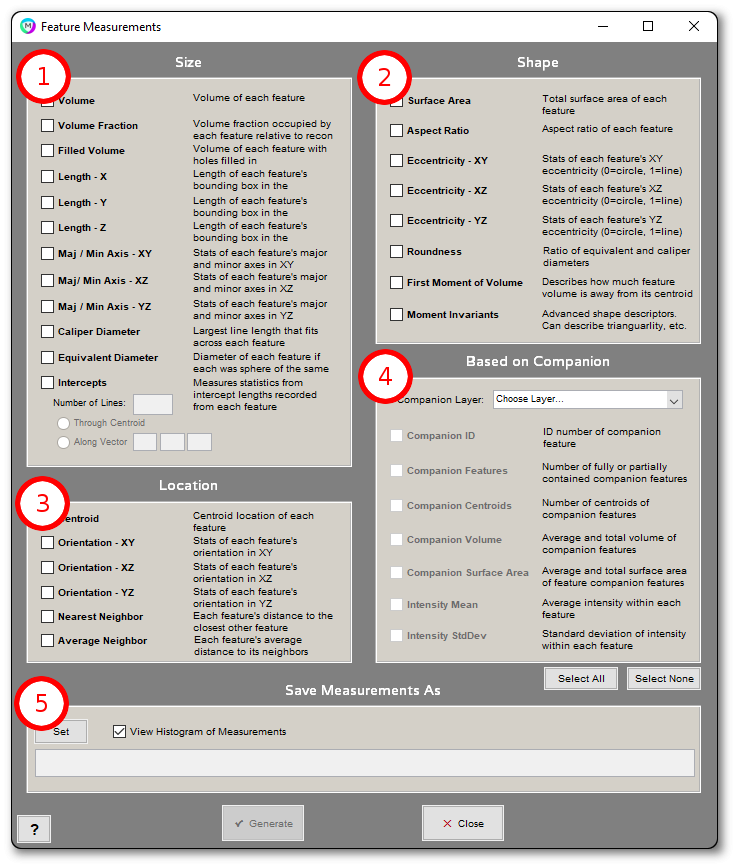### 3D Measurements > Feature Measurements

Feature Measurements allow you to select measurements you would like to generate based on the selected features in the reconstruction.### 1. Size

• Volume: Volume of each feature.
• Volume Fraction: Volume fraction occupied by each feature relative to entire reconstruction volume.
• Filled Volume: Volume of each feature with holes filled in.
• Length – X: Length of each feature’s bounding box in the X-direction
• Length – Y: Length of each feature’s bounding box in the Y-direction.
• Length – Z: Length of each feature’s bounding box in the Z-direction.
• Maj/Min Axis – XY: Stats of each feature’s major and minor axis in the XY plane.
• Maj/Min Axis – XZ: Stats of each feature’s major and minor axis in the XZ plane.
• Maj/Min Axis – YZ: Stats of each feature’s major and minor axis in the YZ plane.
• Equivalent Diameter: Diameter of each feature if each was a sphere of the same volume.
• Caliper Diameter: Largest line length that fits within each feature.
• Intercepts: Measures statistics from intercept length recorded from each feature.
- Through Centroid: Draw each line through each feature’s centroid.
- Along Vector: Draw each line along the same direction through each feature.

### 2. Location

• Centroid: Centroid location of each feature.
• Orientation – XY: Stats of each feature’s orientation in the XY plane, where the angle is calculated by replacing the XY section with an ellipse  and measuring its major axis orientation relative to the X-axis.
• Orientation – XZ: Stats of each feature’s orientation in the XZ plane, where the angle is calculated by replacing the XZ section with an ellipse  and measuring its major axis orientation relative to the X-axis.
• Orientation – YZ: Stats of each feature’s orientation in the YZ plane, where the angle is calculated by replacing the YZ section with an ellipse  and measuring its major axis orientation relative to the Y-axis.
• Nearest Neighbor: Each feature’s distance to the closest other feature, calculated from the features’ centroids.
• Average Neighbor: Each feature’s average distance to its neighbors, as defined by the features’ Delaunay triangulation. Triangulation and distance are both calculated from the features’ centroids.

### 3. Shape

• Surface Area: Total surface area of each feature.
• Aspect Ratio: Magnitude of the largest principal axis, divided by the average of the remaining axes
• Eccentricity – XY: Describes how elongated or circular each feature is in the XY plane per Z section. 0 is a perfect circle. 1 is a straight line.
• Eccentricity – XZ: Describes how elongated or circular each feature is in the XZ plane per Y section. 0 is a perfect circle. 1 is a straight line.
• Eccentricity – YZ: Describes how elongated or circular each feature is in the YZ plane per X section. 0 is a perfect circle. 1 is a straight line.
- Eccentricity is calculated from the equivalent ellipse as sqrt(1-([minor axis length]/[major axis length])^2) 
• First Moment of Volume *: Describes how much volume is extended away from each feature’s centroid. Calculated as the summation of (volume x distance from centroid) over all voxels in the feature.
• Moment Invariants: High-order moments which describe different shape properties of each feature [2,3].

### 4. Based on Layer

• Companion ID: Feature ID of the Companion that contains the Current feature being measured.
• Companion Features: Number of features in the Companion layer that are in the Current layer.
• Companion Centroids: Number of centroids in the Companion layer that are in the Current layer.
• Companion Volume: Average and total volume of features in the Companion layer that is in the Current layer.
• Companion Surface Area: Average total surface area in the Companion layer that is in the Current layer.
• Intensity Mean: Average grayscale intensity within each feature.
• Intensity StdDev: Standard deviation of grayscale intensity within each feature.

### 5. Save Measurement File As

The “Set” button will allow you to choose where you would like the measurement results to be saved. Check “View Histogram of Measurements” to view results as a histogram after generation.

### References

 Ellipse is fit to the feature as the ellipse with the same second moments as the feature. Second moments are calculated as:
uxx = sum(x^2)/N + 1/12
uyy = sum(y^2)/N + 1/12
uxy = sum(x*y)/N

where x is the x coordinate of each pixel in the feature, y is the y coordinate of each pixel, and N is the number of pixels.

The major and minor axes are calculated as:

common = sqrt((uxx – uyy)^2 + 4*uxy^2)
MajorAxisLength = 2*sqrt(2)*sqrt(uxx + uyy + common
MinorAxisLength = 2*sqrt(2)*sqrt(uxx + uyy – common)

 Rosin, Paul L. 2003. “Measuring Shape: Ellipticity, Rectangularity, and Triangularity.” Machine Vision and Applications 14 (3): 172–184.

 MacSleyne, J P, J P Simmons, and M De Graef. 2008. “On the Use of Moment Invariants for the Automated Analysis of 3D Particle Shapes.” Modelling and Simulation in Materials Science and Engineering 16 (4) (June 1): 045008.

Need more help with this?
Chat with an expert now ››